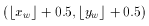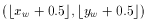# glPointSize

### NAME

glPointSize -- specify the diameter of rasterized points

### C SPECIFICATION

```void glPointSize(GLfloat size)
```

### PARAMETERS

size
Specifies the diameter of rasterized points. The default is 1.0.

### DESCRIPTION

glPointSize specifies the rasterized diameter of both aliased and antialiased points. Using a point size other than 1.0 has different effects, depending on whether point antialiasing is enabled. Point antialiasing is controlled by calling glEnable and glDisable with argument GL_POINT_SMOOTH.

If point antialiasing is disabled, the actual size is determined by rounding the supplied size to the nearest integer. (If the rounding results in the value 0, it is as if the point size were 1.) If the rounded size is odd, then the center point (x, y) of the pixel fragment that represents the point is computed aswhere w subscripts indicate window coordinates. All pixels that lie within the square grid of the rounded size centered at (x, y) make up the fragment. If the size is even, the center point isand the rasterized fragment's centers are the half-integer window coordinates within the square of the rounded size centered at (x, y). All pixel fragments produced in rasterizing a nonantialiased point are assigned the same associated data, that of the vertex corresponding to the point.

If antialiasing is enabled, then point rasterization produces a fragment for each pixel square that intersects the region lying within the circle having diameter equal to the current point size and centered at the point's (xw, yw). The coverage value for each fragment is the window coordinate area of the intersection of the circular region with the corresponding pixel square. This value is saved and used in the final rasterization step. The data associated with each fragment is the data associated with the point being rasterized.

Not all sizes are supported when point antialiasing is enabled. If an unsupported size is requested, the nearest supported size is used. Only size 1.0 is guaranteed to be supported; others depend on the implementation. The range of supported sizes and the size difference between supported sizes within the range can be queried by calling glGet with arguments GL_POINT_SIZE_RANGE and GL_POINT_SIZE_GRANULARITY.

### NOTES

The point size specified by glPointSize is always returned when GL_POINT_SIZE is queried. Clamping and rounding for aliased and antialiased points have no effect on the specified value.

Non-antialiased point size may be clamped to an implementation-dependent maximum. Although this maximum cannot be queried, it must be no less than the maximum value for antialiased points, rounded to the nearest integer value.

### ERRORS

GL_INVALID_VALUE is generated if size is less than or equal to zero.

GL_INVALID_OPERATION is generated if glPointSize is called between a call to glBegin and the corresponding call to glEnd.

### ASSOCIATED GETS

glGet with argument GL_POINT_SIZE
glGet with argument GL_POINT_SIZE_RANGE
glGet with argument GL_POINT_SIZE_GRANULARITY
glIsEnabled with argument GL_POINT_SMOOTH